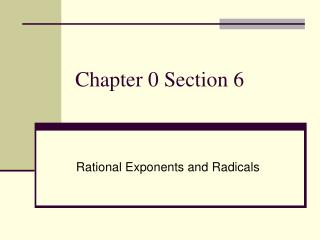DownloadDownload PresentationChapter 0 Section 6

# Chapter 0 Section 6

Download Presentation## Chapter 0 Section 6

- - - - - - - - - - - - - - - - - - - - - - - - - - - E N D - - - - - - - - - - - - - - - - - - - - - - - - - - -
##### Presentation Transcript

1. Chapter 0 Section 6 Rational Exponents and Radicals

2. Radicals and Radical Notation The n is called the “index”—it indicates what root to find. The b is called the radicand– it indicates the number you are trying to find the root of… With radical notation, if n=2 we normally don’t put the 2 in for the index, we just know it as the “square root”. If n=3 we call it the “cube root”…but we must put the 3 in for the index.

3. Evaluating Square Roots

4. Evaluating Cube Roots

5. Summary of evaluating NOT a real number IS a real number

6. Mixed Examples

7. Radicals as Fractional Exponents There is a relationship between radical notation and exponents: The denominator of a fractional exponent is the index. The index becomes a fractional exponent instead. All of the properties of radicals work for fractional exponents too.

8. Convert to radical form and evaluate

9. Properties of Radicals Product property: The root of a product is the product of the roots Quotient property: The root of a quotient is the quotient of the roots Power property: The root of a power is the power of the root

10. Simplify the square roots

11. 4y2 = = 4 3 4    n8 64 m4 m 3 3   y6 64y6 4 = = n2 m4 n8 Simplifying algebraic expressions *You want the exponents of the variables to be a multiple of the index.

12. More simplifying algebraic expressions If the exponents on the variables are not multiples of the index, rewrite as a product so one of the exponents is a multiple of the index.

13.  5 5 3 3 3  3 27  5 27 = = 3 = 3  135 Simplify the expressions Simplest Radical Form…

14. 3 2  3 250 4 4 80 216 16 12 5 18 27 = 12 18 3 = = 6 3 3 3 4 4 4 3 3 80 = = = 2 4 5 5 Evaluate the expressions SOLUTION SOLUTION

15.     2 2 2 2 2 3 3 3 3 3 3 3   3 27 54 – 3 (3 – 1)  – –  2 3 = = = 2 2 = Numeric expressions with +/- Combining Like Radicals…just like with combining like variables, you may combine radical expressions if the index and radicand match. If the index and radicands don’t match after you have simplified, you may not combine the radicals and radicands… they aren’t like.

16. Add or Subtract

17. Rationalizing the Denominator If a reduced fraction still has a radical in the denominator it is not really reduced…there is still some work to do…you need to “rationalize the denominator”, that means make the denominator a Rational number instead of an Irrational number. Think: what times the square root of 7 gives 7…

18. Conjugates If the denominator of the fraction also has an addition or subtraction along with the radical you need to use something called the “conjugate”…some examples of conjugate pairs are listed below. Multiply each pair together (use the same rules for FOIL) and see if you notice something special… When multiplying conjugates, the O and I steps can be skipped (they cancel each other out)

19. Simplify Find the conjugate and multiply numerator and denominator by that conjugate.

20. Simplify

21. Properties